﻿ 粉煤灰掺量对简化权重成熟度法预测准确性的影响 The Amount of Fly Ash Influence to Prediction Accuracy Using the Simplified Weighted Maturity Method

Hans Journal of Civil Engineering
Vol.04 No.03(2015), Article ID:15221,7 pages
10.12677/HJCE.2015.43015

The Amount of Fly Ash Influence to Prediction Accuracy Using the Simplified Weighted Maturity Method

Qiling Luo, Weilun Wang*, Wujian Long

Shenzhen Durability Center for Civil Engineering, Guangdong Provincial Key Laboratory of Durability for Marine Civil Engineering, College of Civil Engineering, Shenzhen University, Shenzhen Guangdong

*通讯作者。

Received: Apr. 18th, 2015; accepted: May 10th, 2015; published: May 14th, 2015ABSTRACT

In this paper, the early compressive strength of fly ash concrete is predicted in the Shenzhen eastern transit expressway connecting line project, using the Simplified Weighted Maturity Method. The result of the study shows that: the mean value (predicted value/measured strength) is 1.00; the C.V is 6.5% and the prediction accuracy is high. Under the condition of W/B 0.45 and cementitious material 400 kg/m3, the slope of the strength prediction formula for different fly ash concrete is same and the intercept is different. When fly ash content increases 10%, the intercept reduces 5 MPa.

Keywords:The Simplified Weighted Maturity Method, Early Compression Strength, Prediction, Fly Ash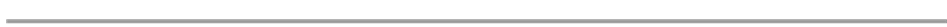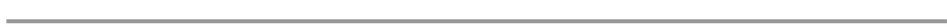1. 前言

2. 简化权重成熟度法

3. 原材料与配合比

4. 试验结果分析Figure 1. The simplified weighted maturity method regression analysisFigure 2. Actual measurement temperature and weighted maturity under the same condition curing of TD-1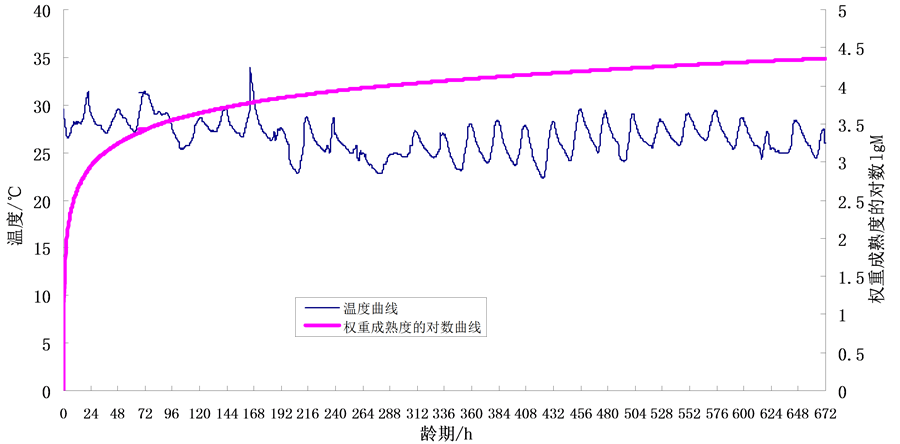Figure 3. Actual measurement temperature and weighted maturity under the same condition curing of TD-2Figure 4. Actual measurement temperature and weighted maturity under the same condition curing of TD-3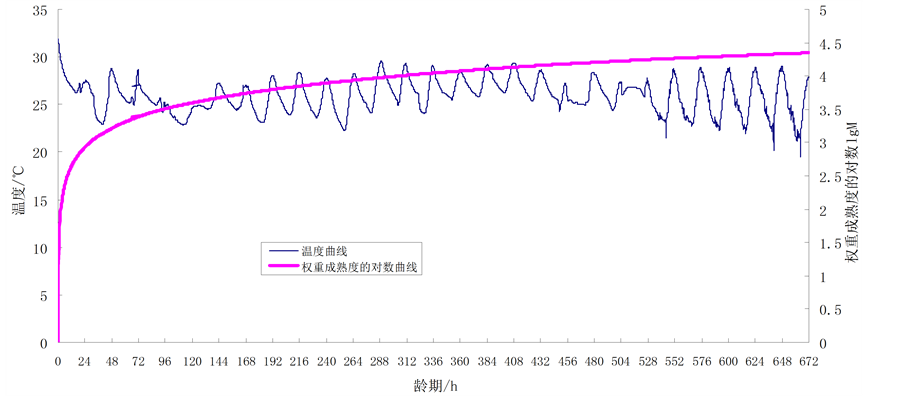Figure 5. Actual measurement temperature and weighted maturity under the same condition curing of TD-4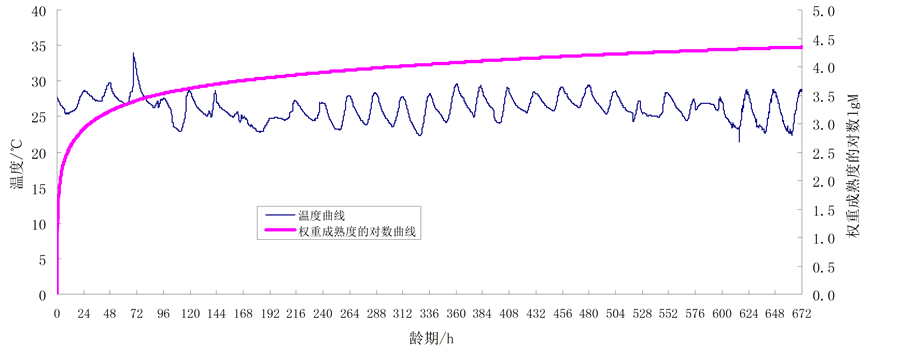Figure 6. Actual measurement temperature and weighted maturity under the same condition curing of TD-6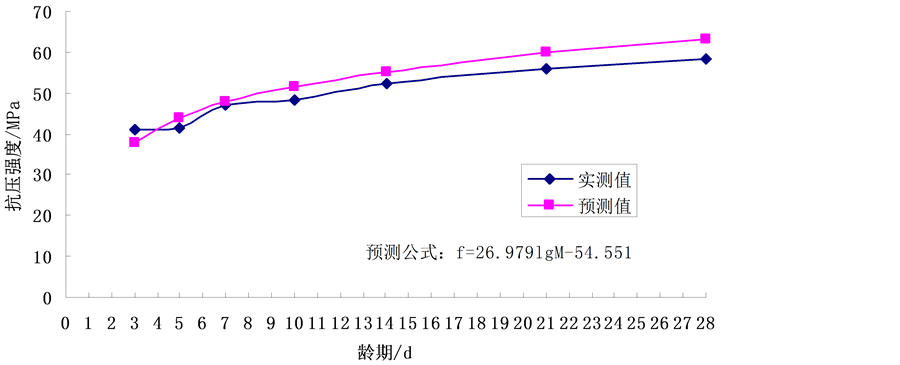Figure 7. Predicted values and measured strength under the same condition curing of TD-1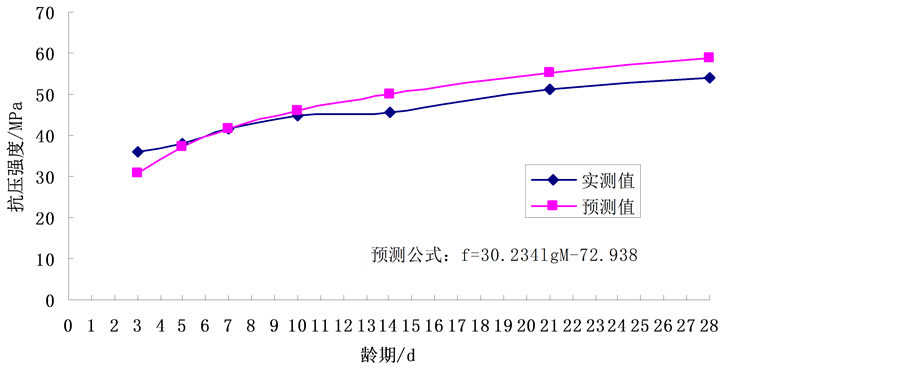Figure 8. Predicted values and measured strength under the same condition curing of TD-2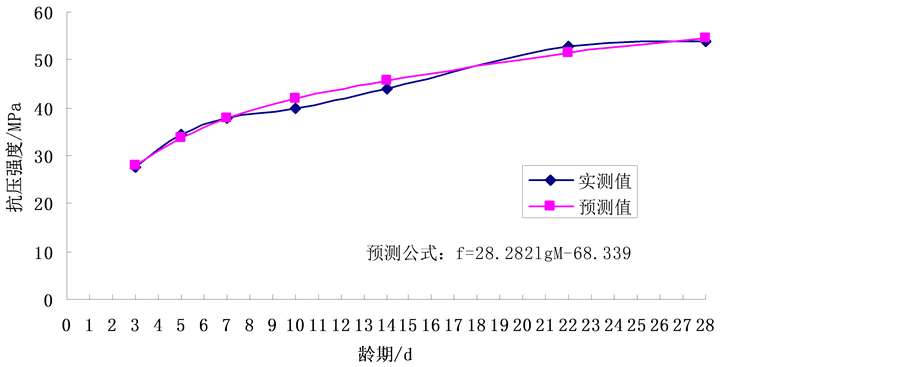Figure 9. Predicted values and measured strength under the same condition curing of TD-3Figure 10. Predicted and measured strength values under the same condition curing of TD-4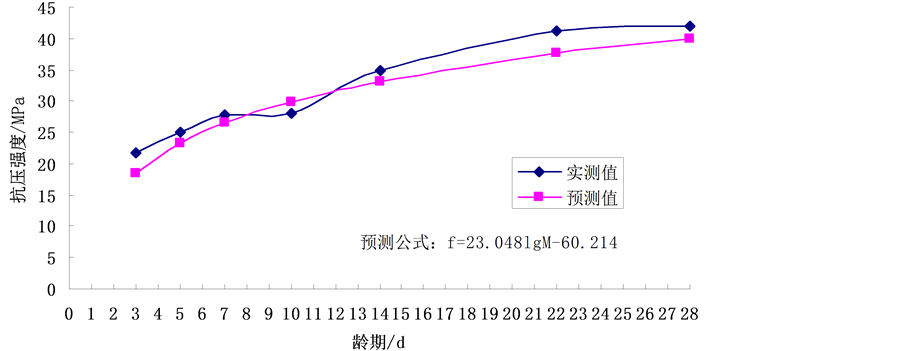Figure 11. Predicted and measured strength values under the same condition curing of TD-5

5. 结论

1) 简化权重成熟度法预测粉煤灰混凝土早期强度，(预测值/实测值)均值1.00，变异系数6.5%，准确性高。Figure 12. The prediction formula of modified interceptTable 2. The (predicted value)/(measured strength) of modified intercept

2) 在水胶比0.45和胶凝材料400 kg/m3固定的情况下，不同粉煤灰掺量的强度预测公式的斜率相同、截距不同；粉煤灰掺量每增加10%，截距减少5 MPa。

The Amount of Fly Ash Influence to Prediction Accuracy Using the Simplified Weighted Maturity Method. 土木工程,03,119-126. doi: 10.12677/HJCE.2015.43015

1. 1. 罗启灵, 邢锋, 韩宁旭, 张志兴, 李雪梅 (2010) 权重成熟度法的合理简化. 混凝土, 4, 34-36.

2. 2. 罗启灵, 邢锋, 韩宁旭, 王玉 (2010) 简化权重成熟度法预测混凝土早期强度. 混凝土, 5, 39-42.